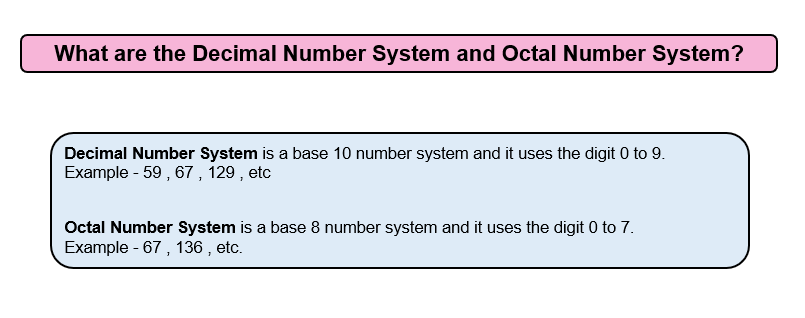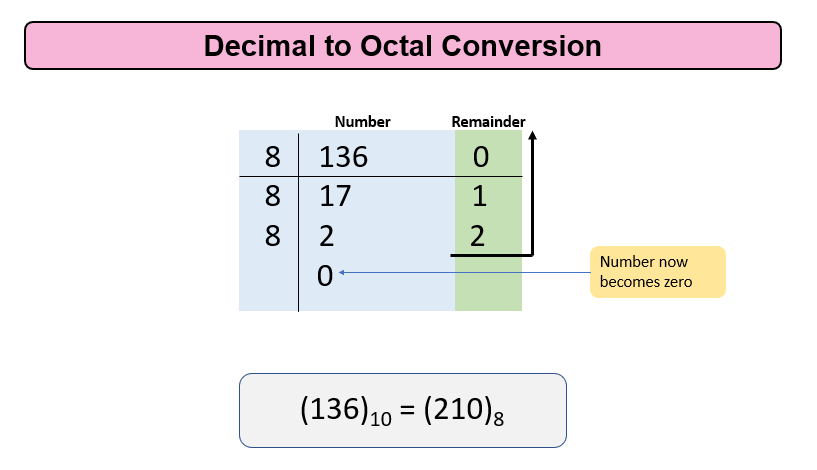# Convert Decimal to Octal

Problem Statement: Given a decimal number, convert it into Octal Number.

Examples:

```Example 1:
Input:  17
Output: 21
Explanation: Octal Equivalent of 17 is 21

Example 2:
Input:  45
Output: 55
Explanation: Octal Equivalent of 45 is 55
```

### Solution

Disclaimer: Don’t jump directly to the solution, try it out yourself first.Approach

For Decimal to Octal Conversion, we will divide the given number by 8 ( Since Octal Numbers System has 8 digits in use ) repeatedly, and its remainder will be stored till the number becomes zero.

Let us take an example for 136

Step 1: Divide 136 by 8, the remainder will be 0 and now n becomes 17.

Step 2: Now divide 17 by 8, the remainder will be 1 so our ans till now becomes 10 and n becomes 2

Step 3: Divide 2 by 8, the remainder will be 2, so the answer till now becomes 210 and n becomes 0

Step 4: since n becomes 0, so print the answer i.e. 210 which is the octal equivalent of the decimal.Code:

## C++ Code

``````#include <iostream>
#include <math.h>
using namespace std;
int DecimaltoOctal(int decimal)
{
int Octal = 0;
int i = 0;
while (decimal != 0)
{
int rem = decimal % 8;
Octal += rem * pow(10, i);
i++;
decimal = decimal / 8;
}
return Octal;
}
int main()
{
int decimal = 136;
cout <<"The Octal conversion of the given decimal number is "<<DecimaltoOctal(decimal) << endl;
return 0;
}
``````

Output:

The Octal conversion of the given decimal number is 210

Time Complexity: O(log n) where n is the number

Space Complexity: O(1)

## Java Code

``````import java.util.*;
public class Main {
public static int DecimaltoOctal(int Decimal) {
int i = 0;
int Octal = 0;
while (Decimal != 0) {
int rem = Decimal % 8;
Octal += rem * Math.pow(10, i);
i++;
Decimal /= 8;
}
return Octal;
}
public static void main(String[] args) {
int Decimal = 136;
System.out.println("The Octal conversion of the given decimal number is
"+DecimaltoOctal(Decimal));
}
}
``````

Output:

The Octal conversion of the given decimal number is 210

Time Complexity: O(log n) where n is the number

Space Complexity: O(1)

Special thanks to Gurmeet Singh for contributing to this article on takeUforward. If you also wish to share your knowledge with the takeUforward fam, please check out this article